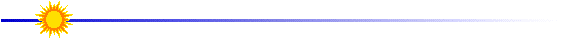# Determining Orbital Velocity

The velocity can be determined once the orbital period is known (see Last Step)  Velocity is defined as the distance an object travels divided by time.  For a circular path, the distance traveled is the circumference, 2pr.  Where "r" is the distance the satellite is from the center of Earth.  Determine the velocity, v  ; be sure you carefully specify your units.

 orbital velocity v = (2p r)  /  t where "t" is orbital period distance from center of Earth r = Eradius + Hheight where "Eradius" = 3960 X 103  m

Determine the orbital velocity of the Yohkoh spacecraft.Lesson designed by the YPOP Team